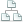Home >Tips >Excel 2016 from Scratch >Calculate Sums, Total, Max and MinSite map

# An Excel tutorial by Peter KalmstromIn this demo in the Excel 2016 from Scratch series Peter Kalmstrom explains how to calculate sum, totals, maximum and minimum.

Peter uses cars as examples. He calculates the total miles for each car per day and week by using AutoSum, and he also finds the total, maximum and minimum miles by using different formulas.

### Exercise

If you want to try the exercise yourself, please download the Excel file Peter uses in the demo.

### Content

This is what Peter shows in the demo below:
• How to calculate the sums of several rows and columns at one time by using AutoSum for the entire range.
• How to select a data range and calculate a total by using a formula.
• How to select a data range and find the maximum miles by using a formula.
• How to select a data range and find the minimum miles by using a formula.
• How to use Cell Styles to differentiate between input cells and calculation cells with formulas.
• How to hold a Ctrl key and select ranges for several separate sets of data.
Peter uses Excel 2016 for his demo, but the Excel basics are the same for earlier versions of Excel.